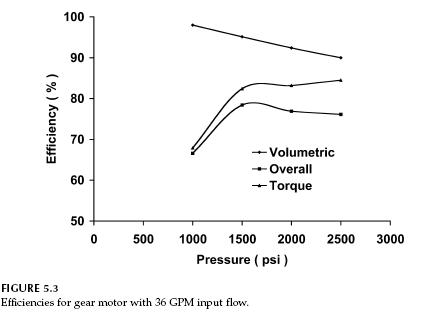﻿ Hydraulic Gear Motor Efficiency – Hydraulic Schematic Troubleshooting

## Hydraulic Gear Motor Efficiency

The gear pump design can also be used as a motor. The Hydreco Model 1919 motor has a theoretical displacement of 4.53 in3/rev, a maximum pressure rating of 2500 psi, and 3000 rpm maximum speed rating. Efficiencies were calculated for a 36 GPM input flow to the motor (Fig. 5.3). Volumetric efficiency decreases linearly as pressure increases. Torque efficiency is almost constant above 1500 psi. Overall efficiency is a maximum at 1500 psi and decreases to 76% at 2500 psi. If the Model 1919 pump and the Model 1919 motor are used together as a hydrostatic transmission, the overall efficiency at 2500 psi (mechanical energy out at the motor divided by mechanical energy in at the pump) would be 62%. No losses in the lines are considered.